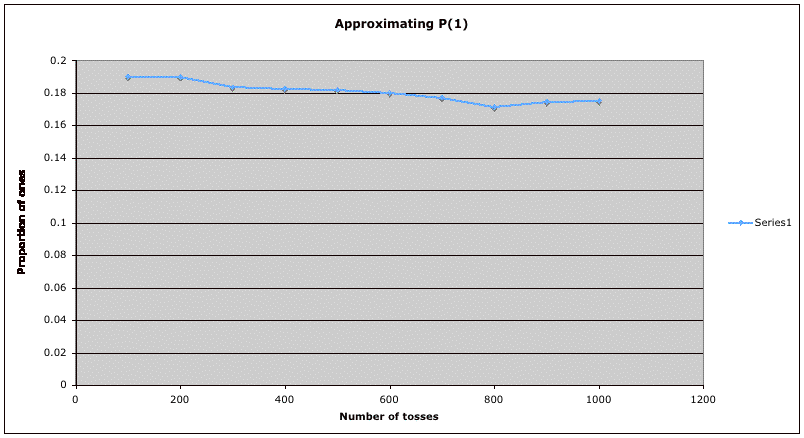## Probability ExampleThis graph illustrates the empirical definition of probability. The horizontal axis shows the number of tosses of a fair die. The vertical axis shows the proportion of those tosses which came up 1.  (These are also shown in the table below.) The trend of the graph is that as the number of tosses increases, the proportion of ones approaches the true probability of 1/6 = 0.16666... . Notice that the zeroing in on the true value is not steady -- in this particular simulation, there is some moving upward from 800 to 1000. However, if we increased the number of tosses to 2000, 3000, etc., we would expect the calculated proportions to  vary less and less from the true value.

 Number of Tosses Proportion of Ones 100 0.19 200 0.19 300 0.18333... 400 0.1825 500 0.182 600 0.18 700 0.177142857 800 0.17125 900 0.1744... 1000 0.175